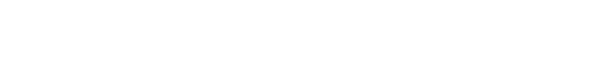# Python when is the function actually executed if using yield

I don’t remember if I have ever used yield keyword in any language in my programming life. When I joined a new project, it is used in the code but I wasn’t sure how it exactly works in Python.

I try to make it clear in this article.

## yield function is not executed immediately

Let’s see a normal function first. If we define the following function, the message is shown on the console when the function is called.

def NonYield():
print("Hello World")

NonYield()
# Hello World

How does it behave if we call the following function?

def DoYield():
print("first")
yield 1
print("second")
yield 2
print("third")
yield 3

print("Call DoYield")
DoYield()
print("End DoYield\n")
# Call DoYield
# End DoYield

As you can see, no print function is called and thus, no message is shown on the console.

## yield function returns a Generator

When yield keyword is used in a function, it returns a Generator.

If we print the return value, we know that the value is a generator.

print("Call DoYield 2")
print(DoYield())
print("End DoYield 2\n")
# Call DoYield 2
# <generator object DoYield at 0x000002B4953ADEB0>
# End DoYield 2

## Generator can be iterated only once

If the object is a Generator, it can be used in for-in but it can be iterated only once.

print("Call DoYield 2")
print(DoYield())
result = DoYield()
[print(v) for v in result]
[print(v) for v in result]
print("End DoYield 2\n")
# Call DoYield 2
# <generator object DoYield at 0x000002221EACCF20>
# first
# 1
# second
# 2
# third
# 3
# End DoYield 2

print is called in the second for-in but the result is not shown because the position in the object has already been forwarded to the end. If it starts from the endpoint, it can do nothing.

## When is the process actually executed

We understand that a function with yield keyword returns a Generator and it can be used in for-in. However, when is the code actually executed?

We confirmed that the code was not executed when the function was called. The code is actually executed when the Generator goes forward.

print("Call DoYield 3-1")
generator = DoYield()
print(next(generator))
print("End DoYield 3-1\n")
# Call DoYield 3-1
# first
# 1
# End DoYield 3-1

The code is partly executed. Let’s check the function again.

def DoYield():
print("first")
yield 1
print("second")
yield 2
print("third")
yield 3

The code until the first yield keyword is added to the Generator. Then, when we call next function again, the message “second” should be shown and 2 is returned as a value.

print("Call DoYield 3-1")
generator = DoYield()
print(next(generator))
print(next(generator))
print("End DoYield 3-1\n")
# Call DoYield 3-1
# first
# 1
# second
# 2
# End DoYield 3-1

What if we call next function more than the length of the Generator?

print("Call DoYield 3-2")
try:
generator = DoYield()
print(next(generator))
print(next(generator))
print(next(generator))
print(next(generator))
except BaseException as err:
print(f"Error occurred: {err=}")
print("End DoYield 3-2\n")

# Call DoYield 3-2
# first
# 1
# second
# 2
# third
# 3
# Error occurred: err=StopIteration()
# End DoYield 3-2

An error is thrown in this case.

In many cases, we don’t have to call next function in the way above. If you just want to call it one by one, use for-in.

print("Call DoYield 4")
for next in DoYield():
print(next)
print("End DoYield 4\n")
# Call DoYield 4
# first
# 1
# second
# 2
# third
# 3
# End DoYield 4

## What if return is used in the same function

Let’s see a case where return and yield are mixed in the same function.

def DoYield2(isInterrupted):
print("first")
yield 1

print("second")
yield 2

if isInterrupted:
return 99

print("third")
yield 3

print("Call DoYield2")
print("When False====")
[print(v) for v in DoYield2(False)]
print("When True====")
[print(v) for v in DoYield2(True)]
print("End DoYield2\n")
# Call DoYield2
# When False====
# first
# 1
# second
# 2
# third
# 3
# When True====
# first
# 1
# second
# 2
# End DoYield2

In this case, if the return is used, the generator stop iterating. Therefore, the third message is not executed.

Let’s see another example. It doesn’t call yield if the argument is true.

def DoYield3(isInterrupted):
print("Start")
if isInterrupted:
return 1
yield 1
yield 2
yield 3

print("Call DoYield3")
result = DoYield3(True)
[print(v) for v in result]
print("End DoYield3")
# Call DoYield3
# Start
# End DoYield3

Even if it doesn’t call yield, the function returns a Generator.

Don’t expect that the function returns int/string value in this case.

Python